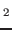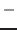Next: Super-cell generation Up: PHON (Version 1.30) A Previous: Calculation of the force

# Description of the program and input/output files

The program reads the following files:

• INPHON: contains the input parameters
• POSCAR: contains the crystal parameters and atomic positions, the format is the same as in the program VASP , and is the following:

SiO2
-47.88
4.2212779176   0.0000000000   0.0000000000
0.0000000000   4.2212779176   0.0000000000
0.0000000000   0.0000000000   2.6869912363
2 4
Direct
0.00000000   0.00000000   0.00000000
0.50000000   0.50000000   0.50000000
0.30657944   0.30657944   0.00000000
0.69342056   0.69342056   0.00000000
0.19342056   0.80657944   0.50000000
0.80657944   0.19342056   0.50000000

This example is the Stishovite structure of SiO. The first line is a comment, the second line is a scale factor, if negative is interpreted as the volume of the system (like in this case). Then the three lattice vectors, followed by the number of atoms for each specie (here 2 silicons and 4 oxygens). The next line precedes the atomic coordinates, and tells if these are in unit of the the lattice vectors of in Cartesian coordinates (here they are in unit of crystal coordinates). Finally the atomic coordinates. Note that the crystal must be in its ground state. It is important to relax the primitive cell very accurately before starting any phonon calculation.

• FORCES: contains the displacements and the force field for each displacement, the format is the following:

4
1  0.005  0.000  0.000
-0.61854500   0.18897800   0.00000000
0.30803200   -0.00013900   0.00000000
0.01746700   0.01833500   0.00000000
-0.01926700   -0.01606600   0.00000000
0.14414800   -0.08517300   0.00000000
0.16816400   -0.10593400   0.00000000
1  0.000  0.000  0.005
0.00000000   0.00000000   -0.29116700
0.00000000   0.00000000   -0.00207200
0.01356000   0.01356000  0.01857500
-0.01356000   -0.01356000   0.01857500
-0.00997600   0.00997600   0.12804500
0.00997600   -0.00997600   0.12804500
3  0.005  0.000  0.000
-0.00548400   -0.00305700   0.00000000
0.15831200   0.09934800   0.00000000
-0.42565500   -0.18284700  0.00000000
0.08338600   0.08818100   0.00000000
-0.01075400   0.01564000   0.00000000
0.20019500   -0.01726600   0.00000000
3  0.000 0.000  0.005
-0.00001000   -0.00001000   0.01963200
0.00388400   0.00388400   0.12906300
0.01036900  0.01036900   -0.25656500
-0.01400200   -0.01400200   -0.00218800
-0.01406100   0.01382000   0.05502900
0.01382000  -0.01406100   0.05502900

The first line is the number of displacements, then, for each displacement, a line containing a number which indicates the position of the atom in the super-cell which has been moved, followed by the displacement (in crystal coordinates), followed by the forces on all the atoms in the super-cell (in units of eV/A and in Cartesian coordinates). For central differences (LCENTRAL = .T.) the format of the file is the same, but there are twice as many displacements (for each displacement u there is alsou).

In this example the super-cell is a primitive cell (to economise on space), in general you need to construct a super-cell, displace the atoms in the primitive cell appropriately and calculate the induced forces. The procedure is explained below.

SubsectionsNext: Super-cell generation Up: PHON (Version 1.30) A Previous: Calculation of the force
Dario Alfe` 2012-02-20